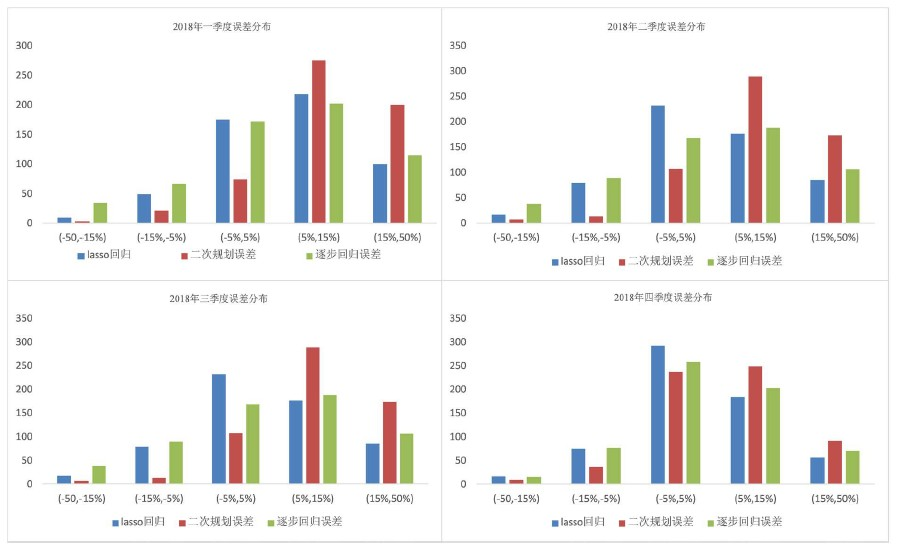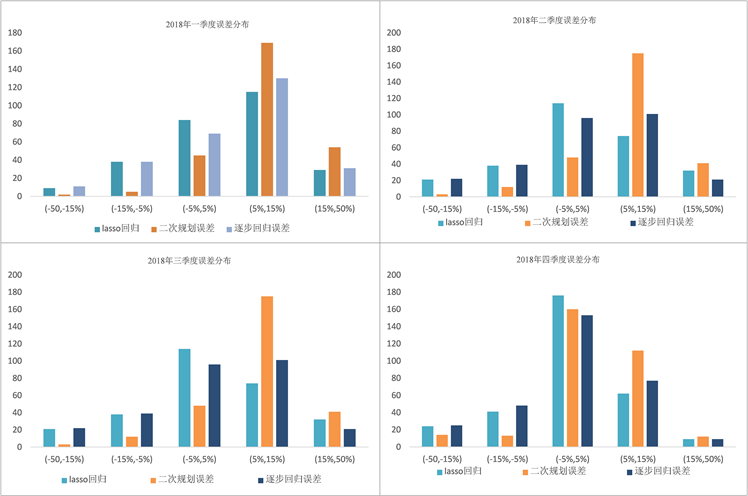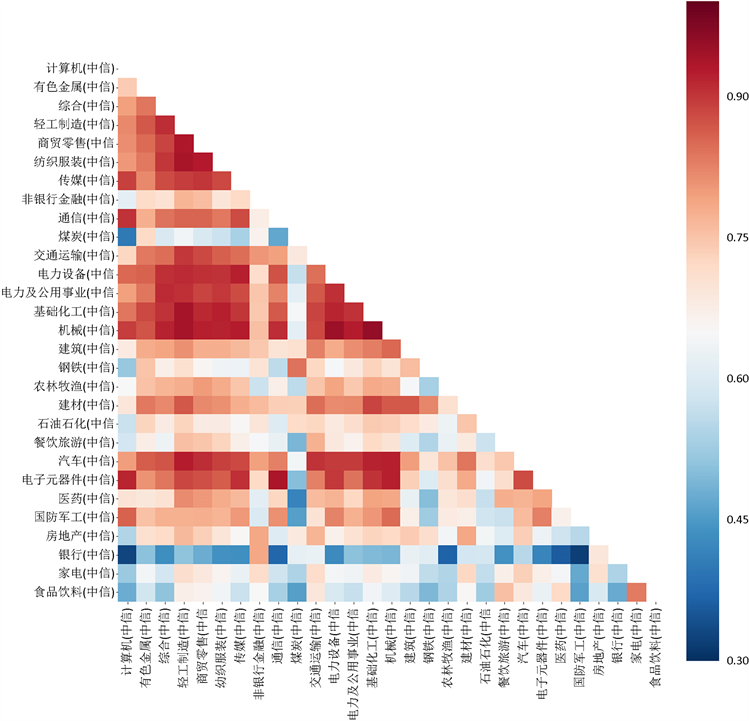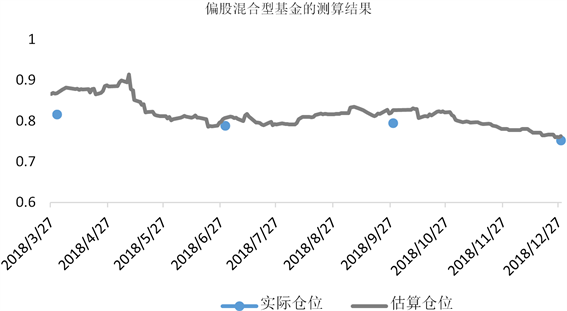# 公募基金仓位测算方法研究Research on the Methods of Estimating the Position of Public Funds

• 全文下载: PDF(1606KB)    PP.307-314   DOI: 10.12677/FIN.2019.94039
• 下载量: 310  浏览量: 857

Fund position is the proportion of funds invested in the stock market to the assets that the fund can use. It’s a reflection of market information and represents investors’ expectations of the market. The position of the fund can be used as an indicator for investors to feel the future trend of the stock market. Public funds only disclose position information at the end of each quarter, which leads to an information asymmetry between investors and fund managers. In the paper, the daily return of public fund and CITIC industry index are taken as dependent and independent variables respectively. The coefficients of industry index are estimated by quadratic programming, stepwise regression and Lasso regression, and then the coefficients are summed up to obtain the position value of the fund. Errors of these three models are compared with the real fund positions published in the quarterly report. The results show that the errors of stepwise regression and Lasso regression are both smaller than that of quadratic programming. Therefore, the two methods are better than quadratic programming in solving such problems.

1. 引言

2. 数据及符号说明

2.1. 数据说明

2.2. 符号说明

3. 多元线性回归模型

${R}_{f,t}={\gamma }_{1}{R}_{1,t}+{\gamma }_{2}{R}_{2,t}+\cdots +{\gamma }_{N}{R}_{N,t}+\epsilon$

4. 二次规划

$\underset{\gamma }{\mathrm{min}}\frac{1}{2T}{\underset{t=1}{\overset{T}{\sum }}\left(\underset{t=1}{\overset{T}{\sum }}{\gamma }_{i}{R}_{i,t}-{R}_{f,t}\right)}^{2}$

${\gamma }_{d}\le \underset{i=1}{\overset{N}{\sum }}{\gamma }_{N}\le {\gamma }_{u}$

$0\le {\gamma }_{i}\le 1,\text{\hspace{0.17em}}\text{\hspace{0.17em}}\text{\hspace{0.17em}}i=1,2,\cdots ,N$Figure 1. Error distribution of partial-equity hybrid fundsFigure 2. Error distribution of common stock fundsFigure 3. Relevance coefficient of industry index

5. 改进的模型

5.1. 逐步回归

5.2. Lasso回归

Lasso回归是引入L1正则化项的线性回归，是多元线性回归的有偏估计，适用于共线性数据，它可以通过将系数进行压缩直到零从而达到剔除部分自变量的目的。Lasso回归的结果对正则化系数λ取值敏感。实际使用过程中，需要通过交叉验证的方法选择合适的λ值。

$J\left(\gamma \right)=\frac{1}{2T}{{\sum }_{t=1}^{T}\left({\sum }_{i=1}^{N}{\gamma }_{i}{R}_{i,t}-{R}_{f,t}\right)}^{2}+\lambda {\sum }_{i=1}^{N}|{\gamma }_{i}|$Figure 4. Position comparison of partial-equity hybrid fundsFigure 5. Position comparison of common stock funds

6. 模型结果对比Table 3. Error comparison of average position

7. 结论

  Markowitz, H.M. (1952) Portfolio Selection. The Journal of Finance, 7, 77-91. https://doi.org/10.1111/j.1540-6261.1952.tb01525.x  Treynor, J.L. (1965) How to Rate Management Investment Funds. Harvard Business Review, 43, 63-75.  Fama, E.F. and French, K.R. (2015) A Five-Factor Asset Pricing Model. Journal of Fi-nancial Economics, 116, 1-22. https://doi.org/10.1016/j.jfineco.2014.10.010  杨艳军, 刘琦. 开放式股票型基金仓位配置模型应用研究[J]. 中南大学学报(社会科学版), 2011, 17(5): 49-54.  何青峰. 探究开放式股票型基金仓位配置模型应用[J]. 中国外资, 2012(24): 24.  马永谙19条微博: 基金仓位测算之我见[J]. 股市动态分析, 2011(23): 66-67.  海琴, 谢怀军. 开放式股票型基金的仓位预测研究[J]. 中国证券期货, 2013(8): 36-37.  曲荣华, 马莉媛, 詹从赞, 童行伟. 基金仓位估算的修匀模型[J]. 数理统计与管理, 2014, 33(5): 932-941.